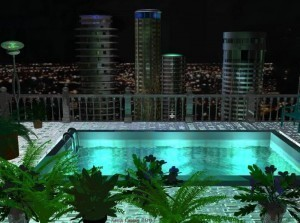# Pool Size Calculator

If you have a pool, knowing how to calculate pool size is a necessity. By knowing how many gallons of water are present, application of cleaning chemicals will be correct. This water volume size is also needed if water testing is to be performed.

### Determine the Average Depth

The first step is to get the average depth of the pool. Get a string and mark it in feet. Fasten it to a heavy object. Now using the feet marker you set, check the pool’s deep and shallow ends. Add these two numbers and then divide the results by two. The result is the average depth. Now you can use the average depth to calculate pool size.

### How to Calculate Rectangular Pool Size

For this pool shape, multiply the width, length, average depth and 7.5 (W x L x D x 7.5). The answer is the total gallons being held in the pool. For instance, if the pool is 30 ft long, 15 ft wide with an average depth of 6 ft, you multiply 30 x 15 x 6 x 7.5. The answer is 20,250 gallons.

### How to Calculate Circular Pool Size

For circular / round pools, you have to multiply the diameter twice and multiply it with the average depth and a 5.9 multiplier. The formula is diameter x diameter x average depth x 5.9. To calculate pool size that is 18 feet in diameter with an average depth of 5 feet, use this: 18 x 18 x 5 x 5.9 = 9,558. It has 9,558 gallons.

### How to Calculate Oval Pool Size

The process here is a bit different. You have to multiply the long and short diameter and the average depth with 5.9 (diameter long x diameter short x average depth x 5.9 = total gallons).

Suppose the pool’s long diameter is 30 ft, the short diameter is 15 ft and the depth average is 6 ft. To get the water volume in gallons, multiply 30 x 15 x 6 x 5.9. The result is 15,930, and that’s the number of gallons in the pool.

### Irregularly Shaped Pools

It is difficult to calculate pool size if the shape is irregular. It is best if you contact a professional or specialist to determine the measurements. The shallow end, deep end, wide point, narrow point etc have to be accounted for.

### Determining the Pool Pump Size

Get the average depth by adding the shallow and deep end and divide it by 2. Multiply the average depth by the width, length and correct multiplier (7.5 for rectangles, 5.9 for ovals and circulars). The result is the gallon total.

To get the flow rate, use the following formula: gallons / hours / 60 minutes. The result is the gallons per minute. This is the amount the pump filter must generate. Now you can get the right filter size for the pool. There are several suppliers and manufacturers that can help you with this.

After you calculate pool size, you can now apply the right chemical cleaning treatments. Follow the instructions as indicated in the package.